# Online Class: Precalculus 101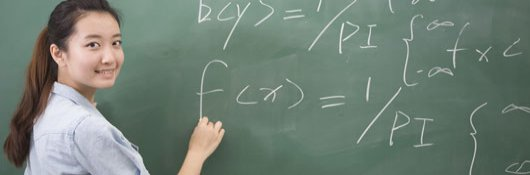\$85.00
no certificate
- OR -
\$110.00
with CEU Certificate*
Start Right Now!
Taking multiple courses? Save with our platinum program.

• 19
Lessons
• 21
Exams &
Assignments
• 35
Hours
average time
• 3.5
CEUs
• 1,669
Students
have taken this course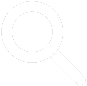## Course Description

Calculus is a mathematical tool that is required in a variety of scientific, technical, and other professional fields. Because it is a higher-level area of math, many people think it is a subject that they can never understand. Unfortunately, such an attitude limits an individual's career choices, particularly in a technology-focused society.

This course provides a bridge between algebra and calculus, guiding students through the underlying mathematical concepts needed to understand and appreciate calculus. The course reviews real and complex numbers, functions, and equations and inequalities. It then goes into some detail on specific types of functions, such as polynomials, exponentials, and logarithms. It also provides a derivation of the principles of trigonometry on the basis of simple right-triangle and circle geometry. After reviewing vectors, polar coordinates, and conic sections, the material sets up calculus proper by examining limits as well as sequences and series.

The final lessons of the course use the basic principles of algebra to derive the two key tools of calculus: differentiation (the derivative) and integration (the integral). At the end of this course, students will be prepared to study calculus in greater depth, further investigating and applying derivatives and integrals to solve a variety of math, physics, and other problems.

• Completely Online
• Self-Paced
• Printable Lessons
• Full HD Video
• 6 Months to Complete
• Start Anytime
• PC & Mac Compatible
• Android & iOS Friendly
• Accredited CEUs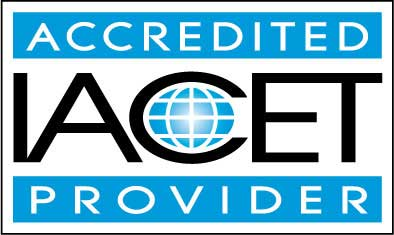## Course Lessons

Average Lesson Rating: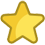#### Lesson 1 - Real and Complex Numbers

In this lesson, we begin the process of setting a foundation for understanding calculus by looking briefly at real and complex numbers. These are the sets of values that we will be studying at length in this course. 21 Total Points
•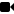Lesson 1 Video A
•Lesson 1 Video B : How to Take an Exam
•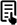Review 3 Articles: Definition of Absolute Value; Interval Notation; Number sense
•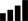Take Survey: Reasons for Taking this Course
•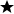Complete Assignment: Introduction
•Complete: Lesson 1 Exam

#### Lesson 2 - Functions I

Having introduced the real and complex number sets in the previous lesson, we now begin to look in depth at series of operations on numbers, or more particularly, numbers and variables. 20 Total Points
•Lesson 2 Video
•Review Article: Independent and Dependent Variables
•Complete: Lesson 2 Exam

#### Lesson 3 - Functions II

Having defined functions in the previous lesson, we now look in more detail at the characteristics of functions. These concepts are critical and will underlie the remainder of this course. 20 Total Points
•Lesson 3 Video
•Review Article: Composition of two functions
•Complete: Lesson 3 Exam

#### Lesson 4 - Equations and Inequalities

Having looked in detail at functions, the course now turns to equations and inequalities: two mathematical expressions that relate different algebraic relations involving numbers, variables, or both. 20 Total Points
•Lesson 4 Video
•Complete: Lesson 4 Exam

#### Lesson 5 - Polynomials and Rational Functions I

Having looked in general at characteristics of functions and their relationship to equations and inequalities, we now turn to a common class of functions, polynomials, and their close cousins, rational functions.x` 20 Total Points
•Lesson 5 Video
•Review Article: Graphing the Rational Function
•Complete: Lesson 5 Exam

#### Lesson 6 - Polynomials and Rational Functions II

In the previous lesson, we defined and discussed some characteristics of polynomials and rational functions. In this lesson, we will look at these functions in slightly more depth. 20 Total Points
•Lesson 6 Video
•Review 2 Articles: How to Solve Higher Degree Polynomials; Rational Functions and Asymptotes
•Complete: Lesson 6 Exam

#### Lesson 7 - Exponential and Logarithmic Functions

This lesson turns away from polynomials (and their close cousins, rational functions) and looks at another type of function: exponential and logarithmic functions. We consider the basic rules of exponents and logarithms and apply them to simple functions. 20 Total Points
•Lesson 7 Video
•Review 2 Articles: LOGARITHMIC AND EXPONENTIAL FUNCTIONS; Solving Equations Using Logarithms
•Complete: Lesson 7 Exam

#### Lesson 8 - Trigonometric Functions I

We now consider another class of functions called trigonometric ("trig") functions. These functions are useful in a variety of contexts involving triangles and circles. This lesson considers the origin of these functions in right triangles and looks at so 20 Total Points
•Lesson 8 Video
•Review 2 Articles: Basic Trigonometry Rules; Right Triangle Trig Calculator
•Complete: Lesson 8 Exam

#### Lesson 9 - Trigonometric Functions II

In the previous lesson, we reviewed the origin of trigonometric functions in the context of right triangles. Interestingly, however, trig functions also have applicability to circles. The present lesson will focus on circles and the relationship of circle 20 Total Points
•Lesson 9 Video
•Review Article: The Trigonometry of Circles
•Complete: Lesson 9 Exam

#### Lesson 10 - Trigonometric Functions III

In this lesson, we will look at a few more aspects of trigonometry, including trigonometric identities and inverse trigonometric functions. 20 Total Points
•Lesson 10 Video
•Review 2 Articles: Inverse Trigonometric Functions; Table of Trigonometric Identities
•Complete: Lesson 10 Exam

#### Lesson 11 - Vectors I

In this lesson, we introduce a new quantity: the vector. Vectors-unlike simple numbers (scalars) that have only a magnitude-have both a magnitude (length) and direction. We will explore how to represent vector quantities, as well as how to add and subtr 20 Total Points
•Lesson 11 Video
•Complete: Lesson 11 Exam

#### Lesson 12 - Vectors II

In the previous lesson, we introduced vectors as quantities with a magnitude and direction. In this lesson, we look more deeply into vectors to flesh out some of the concepts introduced previously. 20 Total Points
•Lesson 12 Video
•Review 3 Articles: Algebraic Representation of Vectors; Unit Vector; Vector Addition and Scalar Multiplication
•Complete: Lesson 12 Exam

#### Lesson 13 - Polar Coordinates

In this lesson, we introduce a new type of coordinate system: polar coordinates. Polar coordinates are one example of the many alternative coordinate systems that can be devised; these alternatives can greatly simplify certain problems. 20 Total Points
•Lesson 13 Video
•Complete: Lesson 13 Exam

#### Lesson 14 - Conic Sections I

In this lesson, we introduce conic sections and show how they are related to cross sections of a cone (hence their name). The basic characteristics-algebraic and graphical-of two conic sections are discussed in detail: circles and parabolas. The next less 20 Total Points
•Lesson 14 Video
•Complete: Lesson 14 Exam

#### Lesson 15 - Conic Sections II

This lesson continues our examination of conic sections, focusing specifically on ellipses and hyperbolas. The end of the lesson presents a practical way of relating all the conics we have studied thus far by way of the motion of celestial objects. 20 Total Points
•Lesson 15 Video
•Review Article: Conic Sections
•Complete: Lesson 15 Exam

#### Lesson 16 - Limits

In this lesson, we introduce limits, a topic fundamental to understanding differentiation and integration-two critical aspects of calculus. Limits are generally intuitive, but a solid ability to use them is important to the remainder of the course. 20 Total Points
•Lesson 16 Video
•Review Article: Basic Limit Laws
•Complete: Lesson 16 Exam

#### Lesson 17 - Sequences and Series

The current lesson switches our focus slightly to look briefly at sequences and series. These mathematical constructs play an important role in calculus and other branches of mathematics. 20 Total Points
•Lesson 17 Video
•Review Article: Introduction to Series
•Complete: Lesson 17 Exam

#### Lesson 18 - Introduction to Derivatives

Having covered all the necessary mathematical background, the final two lessons of the course turn to two fundamental concepts in calculus: the derivative and the integral. Although we will not go into great depth on these subjects, we will show how they 20 Total Points
•Lesson 18 Video
•Review Article: Introduction of Derivatives
•Complete: Lesson 18 Exam

#### Lesson 19 - Introduction to Integrals

In the previous lesson, we covered one of two major aspects of calculus: the derivative. For this closing lesson to the course, we will look briefly at the other of these two concepts: the integral. 100 Total Points
•Lesson 19 Video
•Take Poll: Your Opinion Matters: Course Rating
•Take Survey: Program Evaluation Follow-up Survey (End of Course)
•Complete: Lesson 19 Exam
•Complete: The Final Exam
461
Total Course Points

## Learning Outcomes

By successfully completing this course, students will be able to:
• Define, identify and demonstrate use of real and complex numbers.
• Define, identify and demonstrate use of functions.
• Define, identify and demonstrate use of equations and inequalities.
• Define, identify and demonstrate use of polynomials and rational functions.
• Define, identify and demonstrate use of exponential and logarithmic functions.
• Define, identify and demonstrate use of trigonometric functions.
• Define, identify and demonstrate use of vectors.
• Define, identify and demonstrate use of polar coordinates.
• Define, identify and demonstrate use of conic sections.
• Define, identify and demonstrate use of limits, sequences, and series.
• Define, identify and demonstrate use of derivatives and integrals, and
• Demonstrate mastery of lesson content at levels of 70% or higher.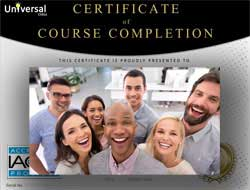• Document Your Lifelong Learning Achievements
• Earn an Official Certificate Documenting Course Hours and CEUs
• Verify Your Certificate with a Unique Serial Number Online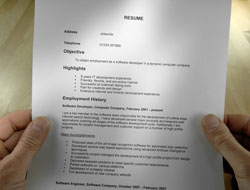Course Title: Precalculus 101
Course Number: 9770456
Languages: English - United States, Canada and other English speaking countries
Category:
Course Type: General Education (Self-Paced, Online Class)
CEU Value: 3.5 IACET CEUs (Continuing Education Units)
CE Accreditation: Universal Class, Inc. has been accredited as an Authorized Provider by the International Association for Continuing Education and Training (IACET).
Assessment Method: Lesson assignments and review exams
Course Author: Dr. Jeffrey Clark, Ph.D.
Instructor: April Cordry-Moore
Syllabus: View Syllabus
Duration: Continuous: Enroll anytime!
Course Fee: \$85.00 (no CEU Certification) || with Online CEU Certification: \$110.00

Course Only
One Course
No Certificate / No CEUs
\$85
for 6 months

Billed once
This course only
 Includes certificate X Includes CEUs X Self-paced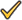Instructor supportTime to complete 6 months No. of courses 1 course
Certificate Course
One Course
Certificate & CEUs
\$110
for 6 months

Billed once
This course only
 Includes certificateIncludes CEUsSelf-pacedInstructor supportTime to complete 6 months No. of courses 1 course
Platinum Yearly
ALL COURSES
Certificates & CEUs
\$189
per year
You save 50%!
Billed once
Includes all 500+ courses
 Includes certificateIncludes CEUsSelf-pacedInstructor supportTime to complete 12 Months No. of courses 500+
Platinum 2 Years
ALL COURSES
Certificates & CEUs
\$299
for 2 years
You save \$427.00!
Billed once
Includes all 500+ courses
 Includes certificateIncludes CEUsSelf-pacedInstructor supportTime to complete 24 Months No. of courses 500+